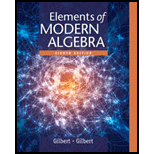# Let S be the set of four matrices S = { I , A , B , C } , where I = [ 1 0 0 1 ] , A = [ 0 − 1 1 0 ] , B = [ − 1 0 0 − 1 ] , C = [ 0 1 − 1 0 ] . Follow the procedure described in Exercise 3 of section 1.4 to complete the following multiplication table for S . (In this case, the product B C = A is entered as shown in the row with B at the left end and in the column with C at the top.) Is S closed under multiplication in M 2 ( ℝ ) ? ⋅ I A B C I A B B C I A C### Elements Of Modern Algebra

8th Edition
Gilbert + 2 others
Publisher: Cengage Learning,
ISBN: 9781285463230### Elements Of Modern Algebra

8th Edition
Gilbert + 2 others
Publisher: Cengage Learning,
ISBN: 9781285463230

#### Solutions

Chapter
Section
Chapter 1.6, Problem 8E
Textbook Problem

## Expert Solution

### Want to see the full answer?

Check out a sample textbook solution.See solution

### Want to see this answer and more?

Experts are waiting 24/7 to provide step-by-step solutions in as fast as 30 minutes!*

See Solution

*Response times vary by subject and question complexity. Median response time is 34 minutes and may be longer for new subjects.